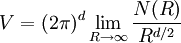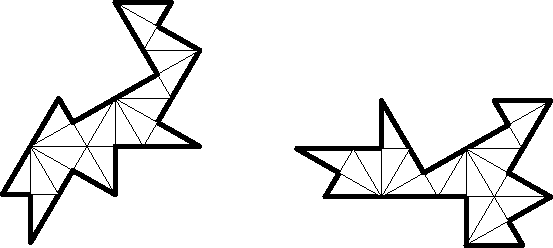# Hearing the shape of a drum

(There appears to be a recent week-long awkward silence on cp4space, partially due to the end of Season II of ciphers. Here’s an attempt to rectify it.)

Suppose we have a square drum, consisting of a membrane fixed at its perimeter. The membrane vibrates according to the wave equation, which is the following partial differential equation:$\nabla^2 u = \dfrac{\partial^2 u}{\partial^2 t}$

The Laplacian ∇²u gives the sum of second partial derivatives of the amplitude u with respect to the x and y axes. (More generally, if the square membrane is replaced by the closure of a different manifold, the Laplacian is the sum of second partial derivatives with respect to an orthonormal basis of tangent vectors.)

Eigenfunctions of the Laplacian correspond to steady states of the wave equation, known as normal modes. The simplest case is a one-dimensional vibrating string, where the normal modes are stationary sinusoidal waves with an integral number of half-periods along the length of the string. In the animation below, the first three modes are shown, together with the arithmetic mean of the first and third (which is also a solution by linearity, but not an eigenfunction therefore not a normal mode):The associated eigenvalues form the spectrum of the drum, about which we can deduce certain properties of the drum. For example, Hermann Weyl, whom you may recognise from such things as Weyl groups and Weyl vectors, discovered a formula for the area (or volume, depending on the dimension of the drum) of the drum based on the asymptotic growth of the eigenvalues.

Specifically, if d is the dimension of the drum and N(R) counts the number of eigenvalues less than R, the volume is given by:Hence, we can determine the size of a drum simply by the spectrum of eigenvalues. Additionally, circular drums have different eigenfunctions (based on Bessel functions) from square drums, and the associated spectrum of eigenvalues is also different. Consequently, basic facts about the shape of the drum can also be deduced. This motivated the following question by Mark Kac, whom you may recognise from such things as Kac-Moody algebras:

Can the shape of a drum be determined by the eigenvalues of the Laplacian?

### Milnor’s 16-dimensional toroidal drums

You may want to read my earlier article about the Leech lattice, if you’re not acquainted with lattices (geometric lattices, not posets). Alternatively, you could acquire a copy of Sphere Packings, Lattices and Groups.

Recall that the eight-dimensional E8 lattice is defined to be the set of all points in$\mathbb{R}^8$ with the following properties:

• Either all coordinates are integers or all coordinates are half-integers;
• The sum of the coordinates is an even integer.

The sixteen-dimensional even unimodular lattice D16+ is constructed in precisely the same way, but in$\mathbb{R}^{16}$. We can obtain another sixteen-dimensional even unimodular lattice, E8², by taking the Cartesian product of the E8 lattice with itself. D16+ and E8² are distinct lattices, but share many properties (such as the same theta series).

Milnor took the quotient of 16-dimensional Euclidean space by each of these lattices, producing two distinct tori with the same set of eigenvalues, and therefore answering Kac’s question in the negative. It would be nice to have an example in two dimensions, as this could be realised as an actual physical drum*.

* Actually, these 16-dimensional quotients of Euclidean space by even unimodular lattices have applications in theoretical physics, where they underpin certain string theories. But a two-dimensional example would still be nice.

### Two-dimensional drums

It took a long time (until 1992) before a pair of indistinguishable drums was discovered in the plane. The original example by Gordon, Webb and Wolpert was quite sophisticated, so we shall instead exhibit a simpler example by Buser, Doyle, Semmler and Conway. Intriguingly, this involves constructing a pair of identical (enantiomorphic) drums in hyperbolic space, which are then ‘flattened out’ into two distinct Euclidean drums with the same spectrum.These hyperbolic drums are two distinct orbifolds for the *424242 hyperbolic symmetry group, which are two distinct index-7 covers of the triangular orbifold for the *444 group. If we find the intersection of all *424242 groups, we obtain an index-168 normal subgroup I of the full *444 symmetry group, with quotient isomorphic to PSL(2,7). The two *424242 groups, when quotiented by I, give two non-conjugate index-7 subgroups of PSL(2,7). These groups are isospectral, which means that the corresponding Euclidean drums (obtained by connecting seven copies of an acute-angled scalene triangle in the natural way) are also isospectral (by a theorem of Sunada).

Using a similar approach, Conway and Doyle found two ‘peacocks’, which are drums which vibrate in the same way when hit at a particular node. This is stricter than having merely the same eigenvalues:The nodes in question are the obvious ones where six triangles (whose disjoint union is an equilateral triangle) meet.

This entry was posted in Uncategorized. Bookmark the permalink.

### 0 Responses to Hearing the shape of a drum

1.Johnicholas says:

Prompted by this blog post, I googled and enjoyed this paper: http://www.cims.nyu.edu/~heilman/papers/Zelditch-%20ExposV3.pdf‎

It claims that we CAN distinguish the shape of drums with no holes, smooth/analytic boundary, and at least one mirror symmetry, which is cool.

2.Unanimous Cowherd says:

One bit of raw LaTeX isn’t rendered, and shouldn’t it say an *index*-168 normal subgroup I?

•apgoucher says:

Yes, you’re correct, of course. I’ll amend it immediately.

3.Nathan Ho says:

This kind of thing is really useful for physical modeling synthesis of realistic drum sounds. I wonder if someone can synthesize a higher-dimensional drum so we can hear what it sounds like.

4.Evelyn Lamb (@evelynjlamb) says:

The AMS Notices just had an article about “hearing the shape of a triangle.” http://www.ams.org/notices/201311/rnoti-p1440.pdf